Question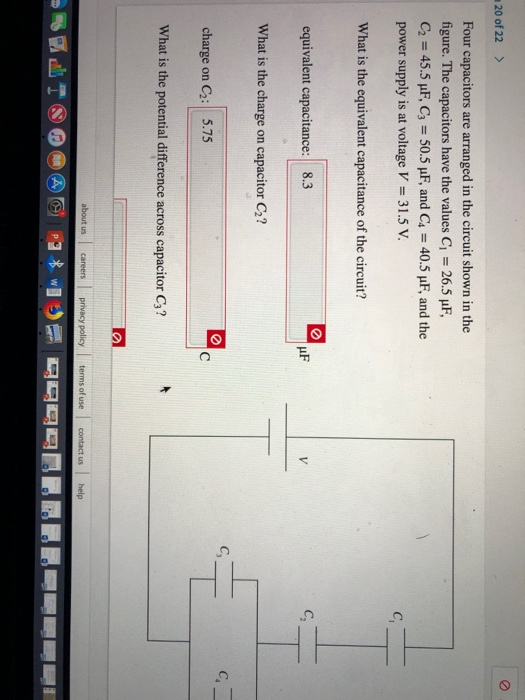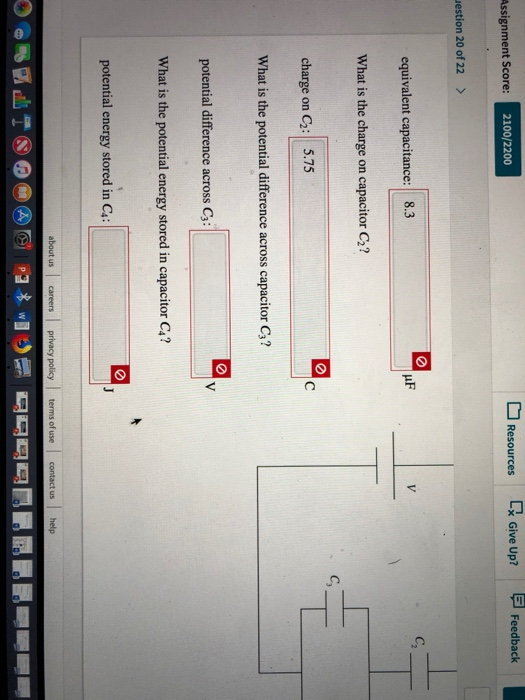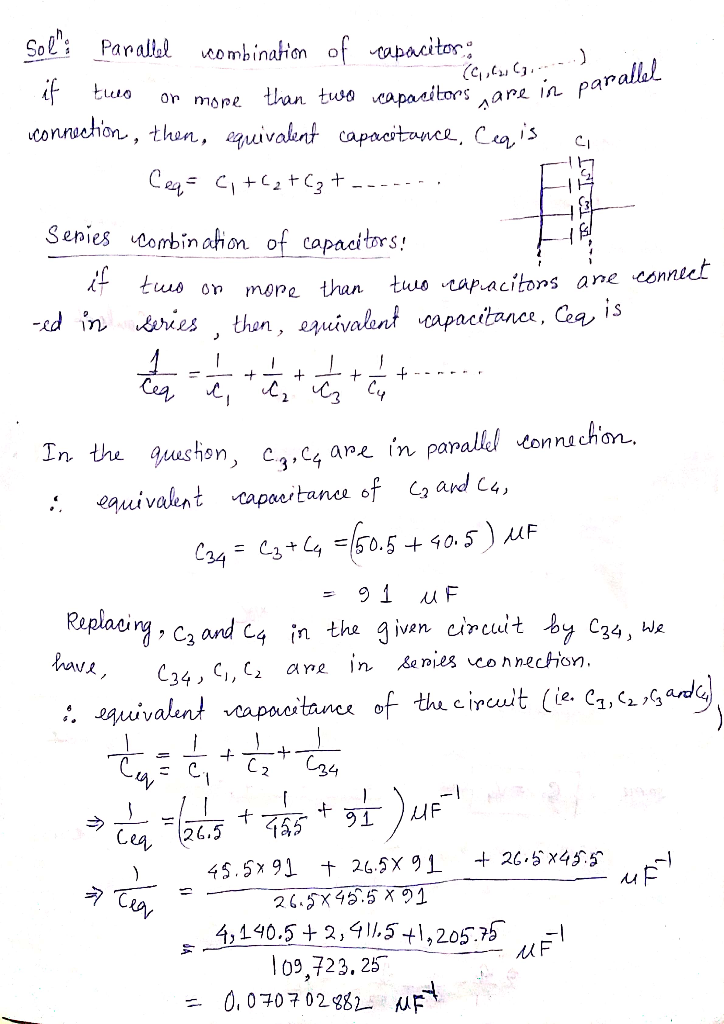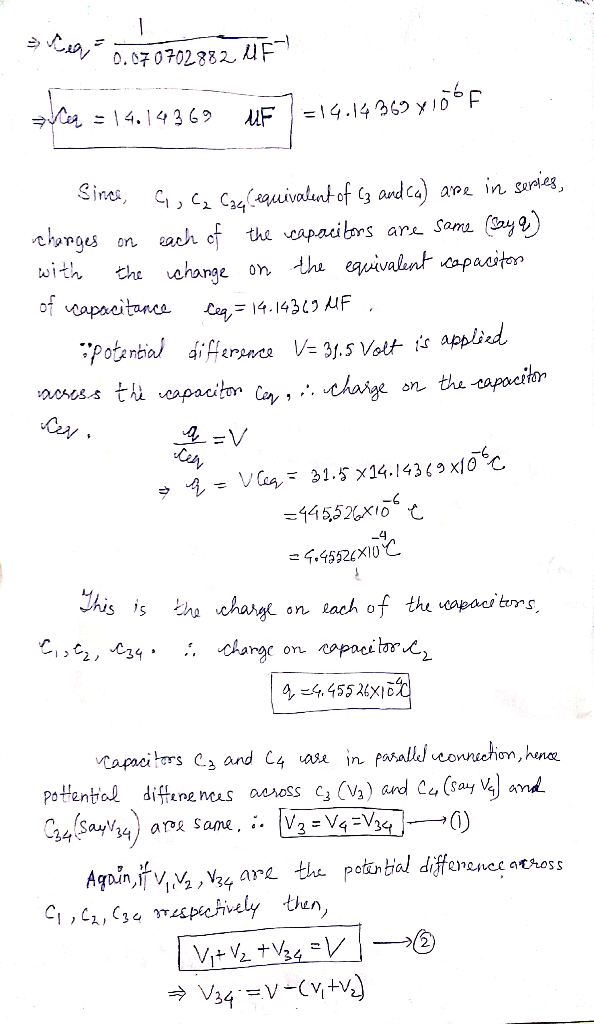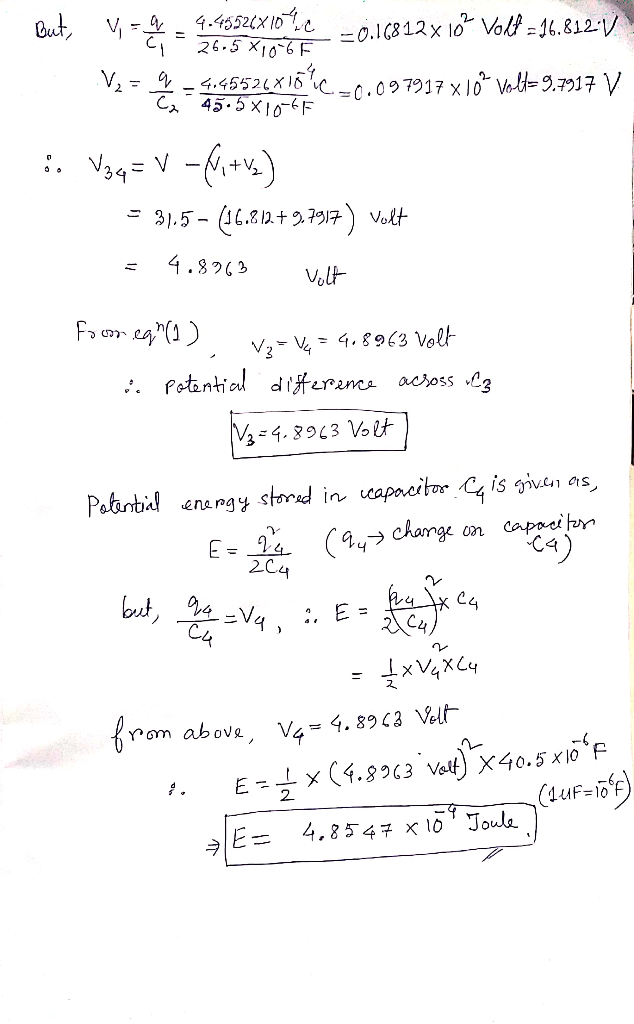#### Earn Coins

Coins can be redeemed for fabulous gifts.

Similar Homework Help Questions
• ### Four capacitors are arranged in the circuit shown in the figure. The capacitors have the values...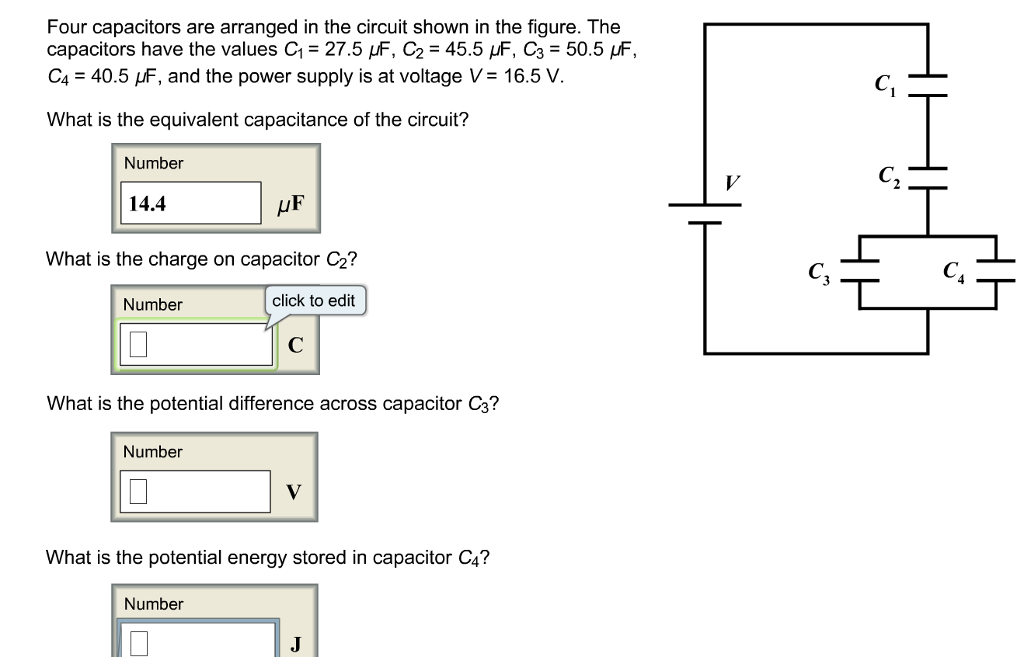Four capacitors are arranged in the circuit shown in the figure. The capacitors have the values C1 = 27.5 μF, C2 = 45.5 μF, C3 = 50.5 μF, C4 = 40.5 μF, and the power supply is at voltage V = 16.5 V. What is the equivalent capacitance of the circuit? Four capacitors are arranged in the circuit shown in the figure. The capacitors have the values C1-27.5 μF, C2 = 45.5 μF, C3 = 50.5 F, C.-40.5 and the...

• ### Four capacitors are arranged in the circuit shown in the figure. The capacitors have the values...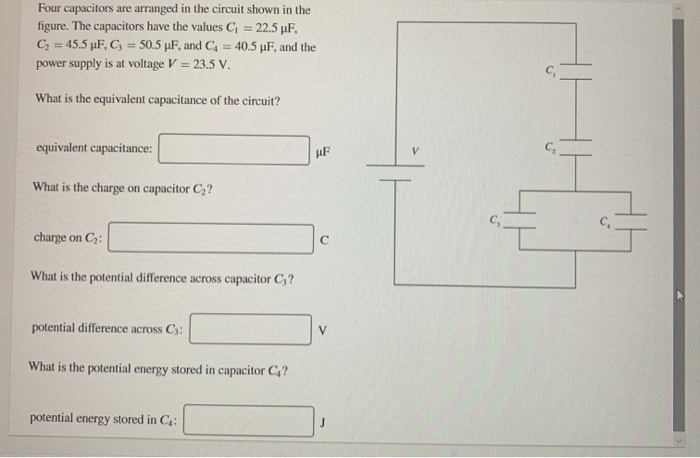Four capacitors are arranged in the circuit shown in the figure. The capacitors have the values G = 22.5 UF, C = 45.5 pF, C; = 50.5 pF, and Cd = 40.5 pF, and the power supply is at voltage V = 23.5 V. What is the equivalent capacitance of the circuit? equivalent capacitance: What is the charge on capacitor C2? charge on C: What is the potential difference across capacitor C;? potential difference across C3: What is the potential...

• ### four capacitors are arranged as in the figure, where C1= 3μF C2= 2μF, C3= 8μF and...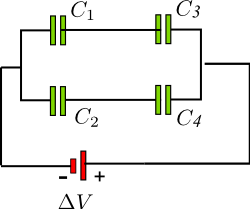four capacitors are arranged as in the figure, where C1= 3μF C2= 2μF, C3= 8μF and C4= 4μF. Potential Difference is ΔV = 90 V Find the equivalent capacitance of the system the charge on C1, the charge on C2 what is the potential Difference across C3, C4?

• ### The circuit in the figure below contains a 90.0 V battery and four capacitors. In the...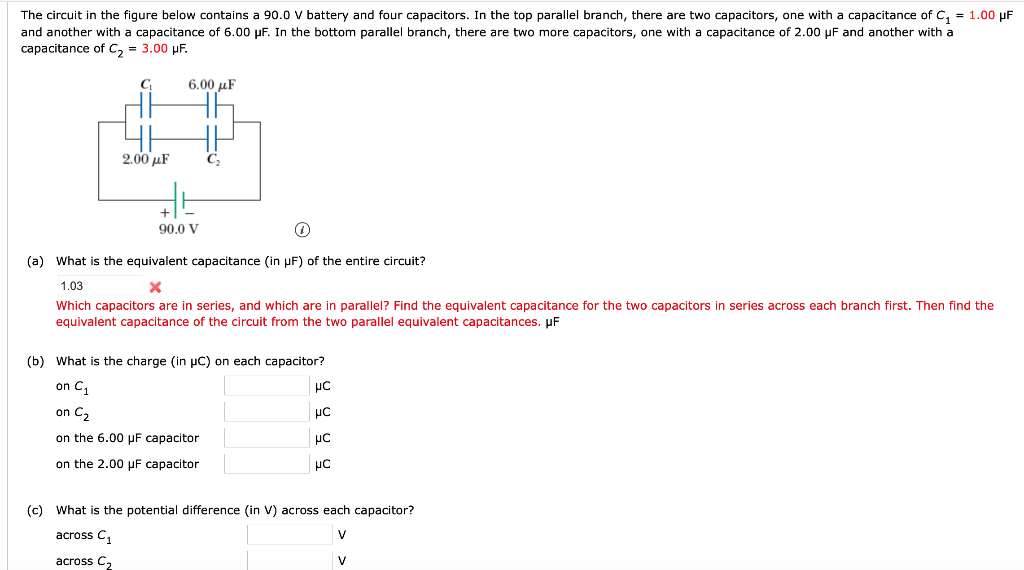The circuit in the figure below contains a 90.0 V battery and four capacitors. In the top parallel branch, there are two capacitors, one with a capacitance of C = 1.00 pF and another with a capacitance of 6.00 pF. In the bottom parallel branch, there are two more capacitors, one with a capacitance of 2.00 pF and another with a capacitance of C2 = 3.00 uF. C 6.00 uF 2.00 uF 90.0 V (a) What is the equivalent capacitance...

• ### A circuit is constructed with five capacitors and a battery as shown. The values for the...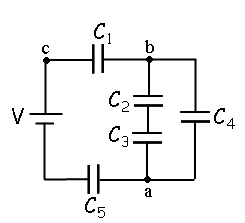A circuit is constructed with five capacitors and a battery as shown. The values for the capacitors are: C1 = C5 = 4.2 μF, C2 = 1 μF, C3 = 5.8 μF, and C4 = 4.4 μF. The battery voltage is V = 12 V. 1) What is Cab, the equivalent capacitance between points a and b?μF 2) What is Cac, the equivalent capacitance between points a and c? 3) What is Q5, the charge on capacitor C5?μC 4) What...

• ### The circuit in the figure below contains a 90.0 V battery and four capacitors. In the...

The circuit in the figure below contains a 90.0 V battery and four capacitors. In the top parallel branch, there are two capacitors, one with a capacitance of C1 = 3.00 µF and another with a capacitance of 6.00 µF. In the bottom parallel branch, there are two more capacitors, one with a capacitance of 2.00 µF and another with a capacitance of C2 = 6.00 µF. A circuit consists of a 90.0 V battery and four capacitors. The wire...

• ### V1 C1 = 3 uF, C2 = 6 F, C3 = 4 uF, C4 = 2uF,...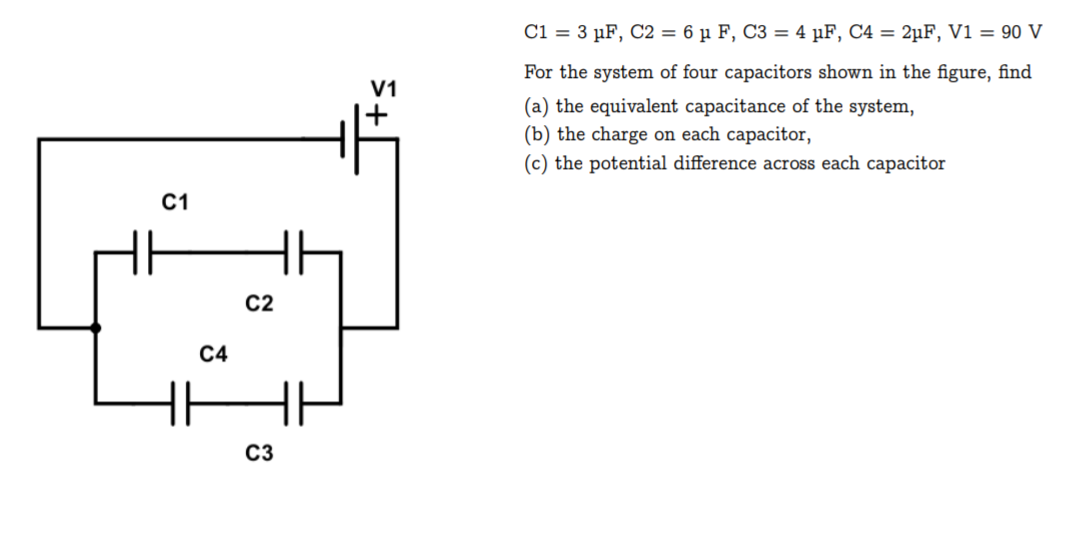V1 C1 = 3 uF, C2 = 6 F, C3 = 4 uF, C4 = 2uF, V1 = 90 V For the system of four capacitors shown in the figure, find (a) the equivalent capacitance of the system, (b) the charge on each capacitor, (c) the potential difference across each capacitor C1 C2 C4 C3

• ### A circuit is constructed with five capacitors and a battery as shown. The values for the...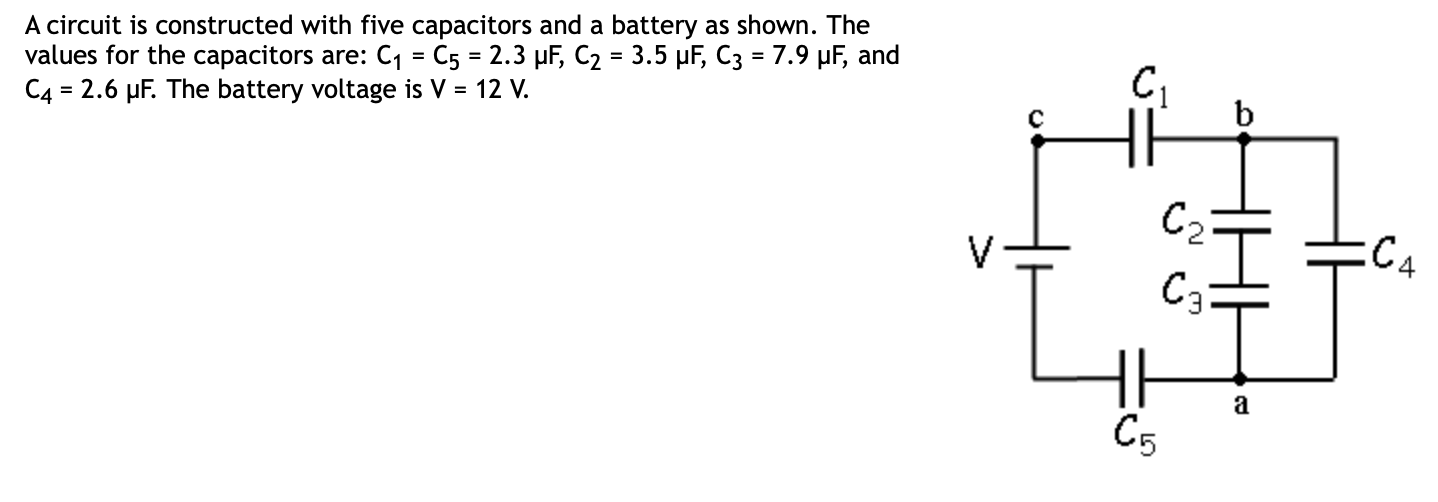A circuit is constructed with five capacitors and a battery as shown. The values for the capacitors are: C1 = C5 = 2.3 uF, C2 = 3.5 uF, C3 = 7.9 pF, and C4 = 2.6 uF. The battery voltage is V = 12 V. EC4 4) What is Q2, the charge on Cz? C Submit 5) What is Q1, the charge on capacitor Cy? uc Submit 6) What is V4, the voltage across capacitor C4? Submit

• ### In the figure (Figure 1), C1 = C5 = 8.3 μF and C2= C3 = C4 = 4.3 μF. The applied potential is Vab = 250 V.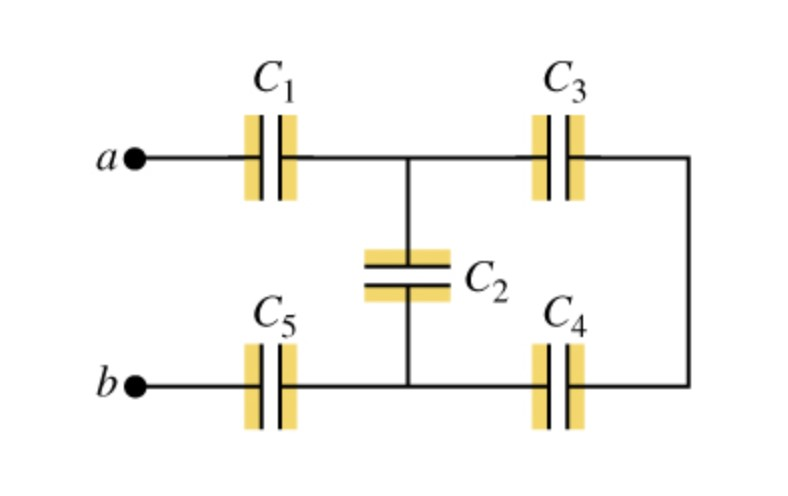In the figure (Figure 1), C1 = C5 = 8.3 μF and C2= C3 = C4 = 4.3 μF. The applied potential is Vab = 250 V.a) What is the equivalent capacitance of the network between points a and b?b) Calculate the charge on capacitor C1.c) Calculate the potential difference across capacitor C1.d) Calculate the charge on capacitor C2.e) Calculate the potential difference across capacitor C2.f) Calculate the charge on capacitor C3.g) Calculate the potential difference across capacitor C3.h) Calculate...

• ### The circuit in the figure below contains a 9.00 v battery and four capacitors. The two...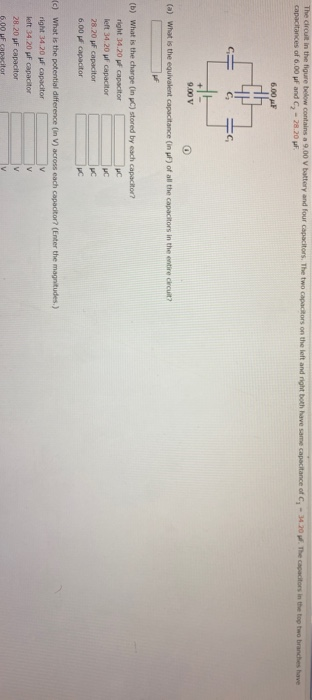The circuit in the figure below contains a 9.00 v battery and four capacitors. The two capacitors on the left and right both have same capacitance of C, -14.20. The capacitors in the top two branches have capacitances of 6.00 pF and C - 28.20 F 6.00 с. 9.00 V (a) What is the equivalent capacitance (in pf) of all the capacitors in the entire circuit? (b) What is the charge (in) stored by each capacitor? right 34.20 pf capacitor...